# Associated Legendre function/Proofs

It is demonstrated that the associated Legendre functions are orthogonal in two different ways and their normalization constant for each is derived.

## Theorem (orthogonality relation 1)$\int\limits_{-1}^{1}P_{l}^{m} \left( x\right) P_{k}^{m} \left( x\right) dx =\frac{2}{2l+1} \frac{\left( l+m\right) !}{\left( l-m\right) !} \delta _{lk},$

where:$P_{l}^{m} \left( x\right) =\frac{\left( -1\right) ^{m} }{2^{l} l!} \left( 1-x^{2} \right) ^{\frac{m}{2} } \frac{d^{l+m} }{dx^{l+m} } \left[ \left( x^{2} -1\right) ^{l} \right], \quad 0\leq m\leq l.$

### Proof

The associated Legendre functions are regular solutions to the associated Legendre differential equation given in the main article. The equation is an example of a more general class of equations known as the Sturm-Liouville equations. Using Sturm-Liouville theory, one can show the orthogonality of functions with the same superscript m and different subscripts:$K_{kl}^{m} =\int\limits_{-1}^{1}P_{k}^{m} \left( x\right) P_{l}^{m} \left( x\right) dx = 0 \quad\hbox{if}\quad k \ne l .$

However, one can find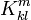$K_{kl}^{m}$ directly from the above definition, whether or not k = l. This involves evaluating the overlap integral directly from the definition of the associated Legendre functions given in the main article. Indeed, inserting the definition of the function twice:$K_{kl}^{m} =\frac{1}{2^{k+l}\; k! \; l! } \int\limits_{-1}^{1} \left\{ (1-x^{2})^{m} \frac{d^{k+m} }{dx^{k+m} } \left[ (x^{2} -1)^{k} \right] \right\} \left\{ \frac{d^{l+m} }{dx^{l+m} } \left[ \left( x^{2} -1\right) ^{l} \right] \right\} dx.$

Since k and l occur symmetrically, one can without loss of generality assume that l ≥ k. Use the well-known integration-by-parts equation$\int_{-1}^1 u\; v'\; dx = \left. u\,v\right|_{-1}^1 - \int_{-1}^{1} v u' \;dx$

l + m times, where the curly brackets in the integral indicate the factors, the first being u and the second v’. For each of the first m integrations by parts, u in the$uv|_{-1}^1$ term contains the factor (1−x2), so the term vanishes. For each of the remaining l integrations, v in that term contains the factor (x2−1) so the term also vanishes. This means:$K_{kl}^{m} =\frac{\left( -1\right) ^{l+m} }{2^{k+l} \; k!\; l! } \int\limits_{-1}^{1}\left( x^{2} -1\right) ^{l} \frac{d^{l+m} }{dx^{l+m} } \left[ \left( 1-x^{2} \right) ^{m} \frac{d^{k+m} }{dx^{k+m} } \left[ \left( x^{2} -1\right) ^{k} \right] \right] dx.$

Expand the second factor using Leibnitz' rule: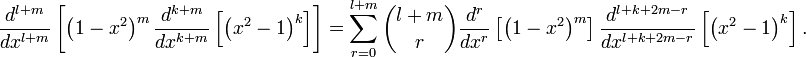$\frac{d^{l+m} }{dx^{l+m} } \left[ \left( 1-x^{2} \right) ^{m} \frac{d^{k+m} }{dx^{k+m} } \left[ \left( x^{2} -1\right) ^{k} \right] \right] =\sum\limits_{r=0}^{l+m} \binom{l+m}{r} \frac{d^{r} }{dx^{r} } \left[ \left( 1-x^{2} \right) ^{m} \right] \frac{d^{l+k+2m-r} }{dx^{l+k+2m-r} } \left[ \left( x^{2} -1\right) ^{k} \right].$

The leftmost derivative in the sum is non-zero only when r ≤ 2m (remembering that ml). The other derivative is non-zero only when k + l + 2mr ≤ 2k, that is, when r ≥ 2m + lk. Because lk these two conditions imply that the only non-zero term in the sum occurs when r = 2m and l = k. So: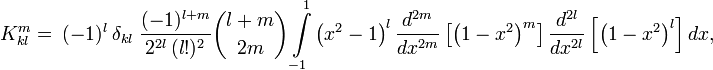$K_{kl}^{m} =\ (-1)^{l}\ \delta_{kl} \; \frac{(-1)^{l+m} }{2^{2l}\, (l!)^{2}} \binom{l+m}{2m} \int\limits_{-1}^{1}\left( x^{2} -1\right) ^{l} \frac{d^{2m} }{dx^{2m} } \left[ \left( 1-x^{2} \right) ^{m} \right] \frac{d^{2l} }{dx^{2l} } \left[ \left( 1-x^{2} \right) ^{l} \right] dx,$

where δkl is the Kronecker delta that shows the orthogonality of functions with lk. The factor ( − 1)l at the front of$K_{kl}^{m}$ comes from switching the sign of x2-1 inside (x2-1)l. To evaluate the differentiated factors, expand (1−x²)k using the binomial theorem: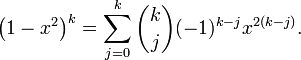$\left( 1-x^{2} \right) ^{k} =\sum\limits_{j=0}^{k} \binom{k}{j} ( -1)^{k-j} x^{2(k-j)}.$

The only term that survives differentiation 2k times is the x2k term, which after differentiation gives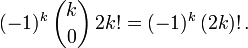$(-1)^k \, \binom{k}{0}\, 2k! = (-1)^{k}\, (2k)! \, .$

Therefore:$K_{kl}^{m} =\ (-1)^{l}\delta _{kl}\; \; \frac{1}{2^{2l}\; (l!) ^{2} } \frac{(2l)!\,(l+m)!}{(l-m)!} \int\limits_{-1}^{1}(x^{2} -1)^{l} dx \qquad\qquad\qquad\qquad\qquad\qquad (1)$

Evaluate$\int\limits_{-1}^{1}(x^{2} -1)^{l} dx$

by a change of variable:$x=\cos \theta\; \Longrightarrow\; dx=-\sin \theta d\theta\quad\hbox{and}\quad 1-x^{2} =(\sin \theta)^2.$

Thus,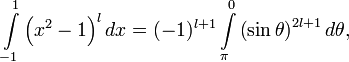$\int\limits_{-1}^{1}\left( x^{2} -1\right) ^{l} dx =(-1)^{l+1}\int\limits_{\pi}^{0}\left( \sin \theta \right) ^{2l+1} d\theta,$

where we recall that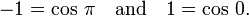$-1 = \cos\,\pi \quad\hbox{and}\quad 1 = \cos\,0.$

The limits were switched from$\pi \rightarrow 0 \; \quad\hbox{and}\quad 0 \rightarrow \pi$ ,

which accounts for one minus sign . Integration of$\frac{d(\sin^{n-1}\theta\cos\theta)}{d\theta} = (n-1)\sin^{n-2}\theta - n\sin^{n}\theta$

gives$\int\limits_{0}^{\pi }\sin^n \theta\; d\theta =\frac{\left. -\sin^{n-1} \theta \cos \theta \right|_{0}^{\pi } }{n} +\frac{(n-1) }{n} \int\limits_{0}^{\pi }\sin ^{n-2} \theta d\theta.$

Since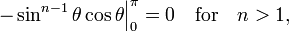$\left. -\sin^{n-1} \theta \cos \theta \right| _{0}^{\pi } =0 \quad\hbox{for}\quad n > 1,$

it follows that$\int\limits_{0}^{\pi }\sin ^{n} \theta d\theta =\frac{\left( n-1\right) }{n} \int\limits_{0}^{\pi }\sin ^{n-2} \theta d\theta$

for n > 1.

Applying this result to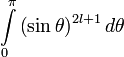$\int\limits_{0}^{\pi }\left( \sin \theta \right) ^{2l+1} d\theta$

and changing the variable back to x yields:$\int\limits_{-1}^{1}\left( x^{2} -1\right) ^{l} dx =\ -\ \frac{2l}{2l+1} \int\limits_{-1}^{1}\left( x^{2} -1\right) ^{l-1} dx$

for l ≥ 1. Using this recursively:$\int\limits_{-1}^{1}\left( x^{2} -1\right) ^{l} dx =\ (-1)^{l}\ (\frac{2l }{2l+1} \frac{2\left( l-1\right) }{2l-1} \frac{2\left( l-2\right) }{2l-3} ...\frac{2}{3} )\ \int\limits_{-1}^{1}\ dx$

Noting: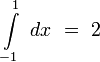$\int\limits_{-1}^{1}\ dx\ =\ 2$

and$\frac{2l }{2l+1} \frac{2\left( l-1\right) }{2l-1} \frac{2\left( l-2\right) }{2l-3} ...\frac{2}{3} \ =\ \frac{2^ll! }{(2l+1)(2l-1)(2l-3)...3}\ =\ \frac{2^ll! }{\frac{(2l+1)! }{2^ll!}}\ =\frac{2^{2l} \left( l!\right) ^{2} }{\left( 2l+1\right) !}$$\int\limits_{-1}^{1}\left( x^{2} -1\right) ^{l} dx = \ (-1)^{l}\ \frac{2^{2l+1} \left( l!\right) ^{2} }{\left( 2l+1\right) !}$

Applying this result to equation (1):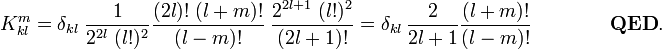$K_{kl}^{m} =\delta _{kl}\; \frac{1}{2^{2l}\; (l!)^{2} } \frac{( 2l)!\;(l+m)!}{(l-m)!}\; \frac{2^{2l+1} \;(l!)^{2} }{( 2l+1)!} = \delta _{kl}\,\frac{2}{2l+1} \frac{( l+m) !}{( l-m) !} \qquad\qquad \mathbf{QED}.$

Clearly, if we define new associated Legendre functions by a constant times the old ones,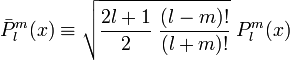$\bar{P}^m_l(x) \equiv \sqrt{ \frac{2l+1}{2}\; \frac{(l-m)!}{(l+m)!} }\; P^m_l(x)$

then the overlap integral becomes,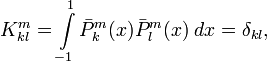$K^m_{kl} = \int\limits_{-1}^{1} \bar{P}^m_k(x) \bar{P}^m_l(x) \;dx = \delta_{kl},$

that is, the new functions are normalized to unity.

The orthogonality of the associated Legendre functions can be demonstrated in different ways. The proof presented above assumes only that the reader is familiar with basic calculus and it is therefore accessible to the widest possible audience. However, as mentioned, their orthogonality also follows from the fact that the associated Legendre equation belongs to a family known as the Sturm-Liouville equations.

It is possible to demonstrate their orthogonality using principles associated with operator calculus. Let us write$P^m_{l}(x) = w(x)^{1/2} \; \nabla^m P_l(x)$

where$\nabla \equiv \frac{d}{dx} \quad \hbox{and}\quad w(x) \equiv (1-x^2)^m.$

Clearly, the case m = 0 is,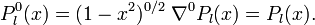$P^{0}_l(x) = (1-x^2)^{0/2}\; \nabla^0 P_l(x) = P_l(x).$

The proof given above starts out by implicitly proving the anti-Hermiticity of ∇. Indeed, noting that w(x) is a function with w(1) = w(−1) = 0 for m ≠ 0, it follows from partial integration that$\langle\; w\, g \;|\; \nabla f\; \rangle \equiv \int\limits_{-1}^1\; w(x)\,g(x)\;\big(\nabla f(x)\big) \; dx = \left. w(x)\;g(x)f(x) \right|_{-1}^{1} - \int\limits_{-1}^1 \Big(\nabla w(x)\,g(x)\Big) \, f(x)\; dx = - \langle\; \nabla (w g) \;|\; f\;\rangle$

Hence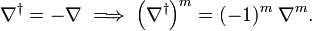$\nabla^\dagger = - \nabla \;\Longrightarrow\; \left(\nabla^\dagger\right)^{m} = (-1)^{m} \;\nabla^{m}.$

To demonstrate orthogonality of the associated Legendre polynomials, we use a result from the theory of orthogonal polynomials. Namely, a Legendre polynomial of order l is orthogonal to any polynomial Πp of order p lower than l. In bra-ket notation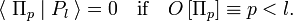$\langle\; \Pi_p \;|\; P_l \;\rangle = 0\quad \hbox{if}\quad O\left[\Pi_p\right] \equiv p < l.$

Knowing this,$\int\limits_{-1}^{1}P_{l}^{m} \left( x\right) P_{k}^{m} \left( x\right) dx \equiv \langle\; w\, \nabla^m P_k \;|\; \nabla^m P_l\;\rangle = (-1)^m \langle\; \nabla^m \{w\, \nabla^m P_k\} \;|\; P_l\;\rangle .$

The bra is a polynomial of order k, because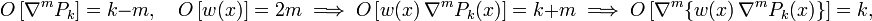$O\left[\nabla^m P_k\right] = k-m, \quad O\left[w(x)\right] = 2m \; \Longrightarrow\; O\left[w(x)\, \nabla^m P_k(x) \right] = k+m \; \Longrightarrow\; O\left[ \nabla^m \{w(x)\, \nabla^m P_k(x)\}\right] = k,$

where it was used that m times differentiation of a polynomial lowers its order by m and that the order of a product of polynomials is the product of the orders. Since we assumed that kl, the integral is non-zero only if k = l. Hence it follows readily that the associated Legendre polynomials of equal superscripts and non-equal subscripts are orthogonal. However, the hard work (given above) of computing the normalization for the case k = l remains to be done.

## Theorem (orthogonality relation 2)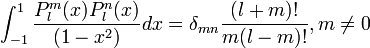$\int_{-1}^{1}\frac{P_{l}^{m}(x) P_{l}^{n} (x)}{(1-x^{2})} dx = \delta_{mn}\frac{(l+m)!}{m(l-m)!} , m \neq 0$.

where: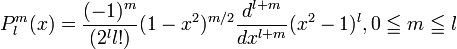$P_{l}^{m}(x) = \frac{(-1)^{m}}{(2^{l} l!)} (1-x^{2})^{m/2} \frac{d^{l+m}}{dx^{l+m}} (x^{2}-1)^{l} , 0 \leqq m \leqq l$.

### Proof

Let: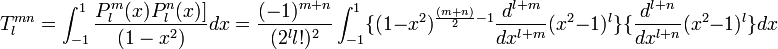$T_{l}^{mn} = \int_{-1}^{1}\frac{P_{l}^{m}(x) P_{l}^{n} (x)]}{(1-x^{2})} dx = \frac{(-1)^{m+n}}{(2^{l} l!)^2} \int_{-1}^{1} \{(1-x^{2})^{\frac{(m+n)}{2}-1} \frac{d^{l+m}}{dx^{l+m}} (x^{2}-1)^{l}\} \{\frac{d^{l+n}}{dx^{l+n}} (x^{2}-1)^{l}\} dx$.

The functions (x2-1)k are even functions; so their jth order derivatives are even or odd functions according as j is even or odd. Therefore, if m or n is even but the other is odd, then one of the two factors in curly braces in the preceding expression is an even function, and the other is an odd function. This makes the entire integrand an odd function. When integrated between the limits that are negatives of each other, it yields 0, as it should, since δmn = 0. So we need to consider further only even integrands, for which m and n are either both even or both odd. In this case all exponents in the integrand are non-negative integers (except when m = n = 0).

The integrand can be integrated by parts$\ell+n$ times, the first factor in curly braces and its derivatives being identified as u and the second factor as v' in the formula:$\int_{-1}^{1} uv' dx = uv \Big|_{-1}^{1} - \int_{-1}^{1} vu' dx$.

Since m and n occur symmetrically, we can assume without loss of generality that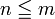$n \leqq m$. For the first n-1 integrations by parts, the uv term vanishes at the limits because u includes at least one factor of (1-x2). The same also is true for the nth integration, unless n = m, in which case the uv term is:$S_{l}^{m} = \frac{(-1)^{m+1}}{(2^{l} l!)^{2}} \frac{d^{m-1}}{dx^{m-1}}\{(1-x^{2})^{m-1} \frac{d^{l+m}}{dx^{l+m}}(x^{2}-1)^{l}\} \{\frac{d^{l}}{dx^{l}}(x^{2}-1)^{l} \} \Big|_{-1}^{1}$.

For the remaining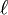$\ell$ integrations the uv term vanishes at the limits because v includes at least one factor (1-x2) (and perhaps u does also). The result is:$T_{l}^{mn} = \delta_{mn}S_{l}^{m} + \frac{(-1)^{l+m}}{(2^{l} l!)^{2}} \int_{-1}^{1} (x^{2}-1)^{l} \frac{d^{l+n}}{dx^{l+n}}\{(1-x^{2})^{\frac{(m+n)}{2}-1} \frac{d^{l+m}}{dx^{l+m}}(x^{2}-1)^{l}\} dx$.

The highest power of x in the binomial expansion of (x2-1)l is$2\ell$; after$\ell+m$ derivatives of it are taken, the highest power is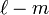$\ell-m$. The highest power of x in the expansion of$(1-x^{2})^{\frac{(m+n)}{2}-1}$ is m+n-2; so the highest power in the expression in curly braces is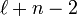$\ell+n-2$. After$\ell+n-2$ derivatives of it are taken, the highest power is 0; that is, the expression is a constant. Taking the remaining 2 derivatives causes the integrand to vanish. Therefore:$T_{l}^{mn} = \delta_{mn}S_{l}^{m} = \delta_{mn}\frac{1}{(2^{l} l!)^{2}} \frac{d^{m-1}}{dx^{m-1}}\{(x^{2}-1)^{m-1} \frac{d^{l+m}}{dx^{l+m}} (x^{2}-1)^{l}\} \{\frac{d^{l}}{dx^{l}}(x^{2}-1)^{l}\} \Big|_{-1}^{1}$.

Since this function is odd, we need evaluate it only at its upper limit x = 1 and double the result.

An expression of the form (x2 − 1)k = (x − 1)k(x + 1)k can be differentiated using Leibnitz's rule. Only one term in the sum is of interest here, namely the one in which (x-1)k is differentiated exactly k times so that no factors of (x-1) remain. If it is differentiated fewer times, then it vanishes at x = 1. If it is differentiated more times, then it vanishes everywhere. So, ignoring terms with factors of (x-1), we have (from right to left in the preceding expression):$\frac{d^{l}}{dx^{l}}(x^{2}-1)^{l} = [\frac{d^{l}}{dx^{l}}(x-1)^{l}](x+1)^{l} = l! (x+1)^{l}$ , which equals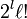$2^{l} \ell!$ when x = 1:$\frac{d^{l+m}}{dx^{l+m}}(x^{2}-1)^{l} = \frac{(l+m)!}{l!m!} [\frac{d^{l}}{dx^{l}} (x-1)^{l}] [\frac{d^{m}}{dx^{m}}(x+1)^{l}]$$= \frac{(l+m)!}{l!m!} [l!] [\frac{l!}{(l-m)!} (x+1)^{l-m}]$$= (l+m)! \frac{l!}{m!(l-m)!} (x+1)^{l-m}$$\frac{d^{m-1}}{dx^{m-1}}\{(x^{2}-1)^{m-1} \frac{d^{l+m}}{dx^{l+m}}(x^{2}-1)^{l}\}$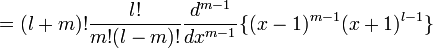$= (l+m)! \frac{l!}{m!(l-m)!} \frac{d^{m-1}}{dx^{m-1}}\{(x-1)^{m-1}(x+1)^{l-1}\}$$= (l+m)! \frac{l!}{m!(l-m)!} (m-1)! (x+1)^{l-1}$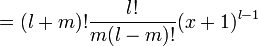$= (l+m)! \frac{l!}{m(l-m)!} (x+1)^{l-1}$ , which equals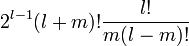$2^{l-1}(l+m)! \frac{l!}{m(l-m)!}$ when x = 1.$T_{l}^{mn} = 2\delta_{mn}\frac{1}{(2^{l} l!)^{2}} \{2^{l-1}(l+m)! \frac{l!}{m(l-m)!}\} \{2^{l} l!\} = \delta_{mn}\frac{(l+m)!}{m(l-m)!}$

which has been doubled to include the lower limit x = -1. QED.

When n = m = 0: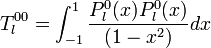$T_{l}^{00} = \int_{-1}^{1} \frac{P_{l}^{0}(x) P_{l}^{0} (x)}{(1-x^{2})} dx$$= \frac{1}{(2^{l} l!)^{2}} \int_{-1}^{1} \{(1-x^{2})^{-1} \frac{d^{l}}{dx^{l}}(x^{2}-1)^{l}\} \{\frac{d^{l}}{dx^{l}}(x^{2}-1)^{l}\} dx$$= \frac{1}{(2^{l} l!)^{2}} \int_{-1}^{1} \frac{1}{2}[(x+1)^{-1} - (x-1)^{-1}] \{\frac{d^{l}}{dx^{l}}(x^{2}-1)^{l}\}^{2} dx$.

When expanded in powers of (x+1) and (x-1) by using Leibnitz's rule, the expression in curly braces includes exactly one term, (x-1)l, having no factor (x+1) and exactly one term, (x+1)l, having no factor (x-1). Therefore in a Laurent series expansion of the integrand about either x = 1 or x = -1 all terms except one have non-negative exponents, and their integral is finite. The one remaining term in the integrand is a constant multiple of 1/(x+1) or 1/(x-1); so its integral logarithmically diverges at the limits, making T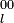$_{l}^{00}$ infinite.Some content on this page may previously have appeared on Citizendium.